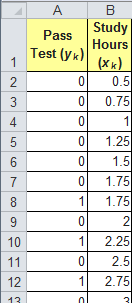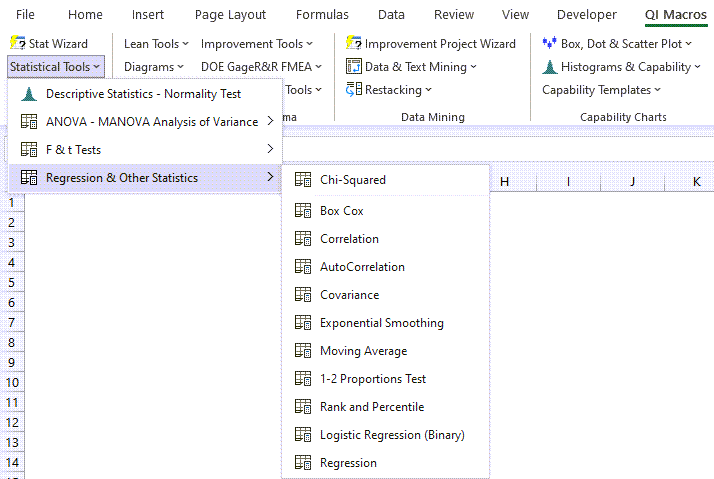# Binary Logistic Regression in Excel using QI Macros

Binary Logistic Regression estimates the probability of an event occurring, such as voted or didn't vote, based on a given dataset of independent variables. Since the outcome is a probability, the dependent variable is bounded between 0 and 1.## How to Conduct Binary Logistic Regression in Excel using QI Macros

Here is an example of Binary Logistic Regression in Excel using QI Macros.

1. Select two or more columns of data (NOTE: The first column of your data must be setup as 1 (pass) or 0 (fail), while the next column(s) in your data set should include your predictors):
2.The sample data shown above is found in QI Macros Help > Open QI Macros Sample Data > statistical.xlsx > Logistic Regression tab

3. Next, click on the QI Macros menu and choose Statistical Tools > Regression & Other Statistics > Logistic Regression (Binary):
4.Excel's Solver add-in is used to perform the Coefficients calculations.

If Solver is not enabled in your Excel, you will be provided with information about how to calculate the Coefficients manually, using Solver.

5. Evaluate the Logistic Regression Results:If you are using a Mac, you will need to calculate the Coefficients manually with Solver.

In this example:

• The probability of passing the test is derived from past data.
• The number of hours of study can then be used to predict the probability that a student will pass the test.

NOTE: We only offer Binary Logistic Regression. We do not offer Multinomial or Ordinal Logistic Regression.

*Functionality introduced in the July 2022 version of QI Macros*

### Why Choose QI Macros Statistical Software for Excel?### Affordable

• Only \$329 USD - less with quantity discounts
• No annual fees
• Free Technical Support### Easy to Use

• Works Right in Excel
• Interprets Results for You
• Accurate Results Without Worry### Proven and Trusted

• 100,000 Users in 80 Countries
• Celebrating 20th Anniversary
• Five Star CNET Rating - Virus Free